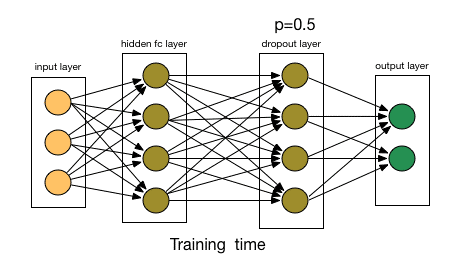/  Deep Learning Interview questions and answers   /  What are the Regularization techniques used in Neural Network?## What are the Regularization techniques used in Neural Network?

Ans: Regularization is a technique which makes slight modifications to the learning algorithm such that the model generalizes better. This in turn improves the model’s performance on the unseen data as well. In deep learning, regularization penalizes the weight matrices of the nodes.

L2 & L1 regularization

L1 and L2 are the most common types of regularization. These update the general cost function by adding another term known as the regularization term.

Cost function = Loss (say, binary cross entropy) + Regularization term

Due to the addition of this regularization term, the values of weight matrices decrease because it assumes that a neural network with smaller weight matrices leads to simpler models. Therefore, it will also reduce overfitting to quite an extent.

However, this regularization term differs in L1 and L2.

In L2, we have:Here, lambda is the regularization parameter. It is the hyperparameter whose value is optimized for better results. L2 regularization is also known as weight decay as it forces the weights to decay towards zero (but not exactly zero).

In L1, we have:In this, we penalize the absolute value of the weights. Unlike L2, the weights may be reduced to zero here. Hence, it is very useful when we are trying to compress our model. Otherwise, we usually prefer L2 over it.

In keras, we can directly apply regularization to any layer using the regularizers.

Dropout

This is the one of the most interesting types of regularization techniques. It also produces very good results and is consequently the most frequently used regularization technique in the field of deep learning.

To understand dropout, let’s say our neural network structure is akin to the one shown below:At every iteration, it randomly selects some nodes and removes them along with all of their incoming and outgoing connections.So, each iteration has a different set of nodes and this results in a different set of outputs. It can also be thought of as an ensemble technique in machine learning.

Ensemble models usually perform better than a single model as they capture more randomness. Similarly, dropout also performs better than a normal neural network model.

So, each iteration has a different set of nodes and this results in a different set of outputs. It can also be thought of as an ensemble technique in machine learning.

Ensemble models usually perform better than a single model as they capture more randomness. Similarly, dropout also performs better than a normal neural network model.Due to these reasons, dropout is usually preferred when we have a large neural network structure in order to introduce more randomness.